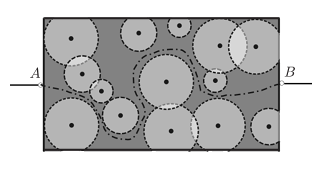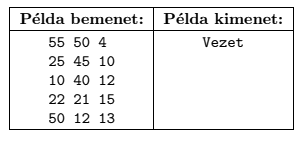Mathematical and Physical Journal
for High Schools
Issued by the MATFUND Foundation
 Already signed up? New to KöMaL?

#Problem I/S. 1. (September 2015)

I/S. 1. A low-voltage circuit contains a thin metal plate, and a machine removes certain disks from this plate. Your task in this exercise is to decide whether the circuit will still be closed after the removal of the disks, that is, whether there is a path between points $\displaystyle A$ and $\displaystyle B$.The metal plate is an $\displaystyle N\times M$ rectangle ($\displaystyle 10\le N,M\le 1000$, with $\displaystyle N$ and $\displaystyle M$ specifying its width and height, respectively). The machine cuts out $\displaystyle K$ disks ($\displaystyle 0\le K\le 100$), and it is possible for the disks to intersect one another. The center of the $\displaystyle i^\mathrm{th}$ disk is given by ($\displaystyle x_{i}$, $\displaystyle y_{i}$) with some integer coordinates within the rectangle, while its radius is $\displaystyle r_i$ ($\displaystyle 0 < r_{i} \le \min(N,M)$ with $\displaystyle r_i$ being some integer).

Points $\displaystyle A$ and $\displaystyle B$ are connected to the rectangle through the complete vertical segments $\displaystyle x=0$ and $\displaystyle x=N$, respectively. When a disk is removed, its interior and boundary are all deleted, so if two disks touch each other, for example, then their point of contact is also removed from the plate.

Your program should read the values of $\displaystyle N$, $\displaystyle M$ and $\displaystyle K$ from the first line of the standard input, then the coordinates of the disk centers and the radii (positive integers) from the next $\displaystyle K$ lines. The first and only line of the standard output should be either ''Conducts'' or ''Does not conduct'', depending on whether or not electricity can pass through the circuit after the removal of the disks.

In the example, Példa bemenet'' is a sample input, while Példa kimenet'' is the corresponding output.Scoring and bounds. You can get 1 point for a brief and proper documentation clearly describing your solution. Nine further points can be obtained provided that your program solves any valid input within 1 second of running time.

The source code of your program with a short documentation of your solution, and also describing which developer environment to use for compiling the source, should be submitted in a compressed file is1.zip.

(10 pont)

Deadline expired on October 12, 2015.

### Statistics:

 24 students sent a solution. 10 points: Csenger Géza, Fuisz Gábor, Gáspár Attila, Hornák Bence, Janzer Orsolya Lili, Kiss Gergely, Mernyei Péter, Nagy Nándor, Németh 123 Balázs, Németh 729 Gábor, Noszály Áron, Palánki Lajos, Uzonyi 000 Ákos, Zarándy Álmos. 9 points: Gergely Patrik. 6 points: 1 student. 3 points: 2 students. 2 points: 4 students. 0 point: 2 students.

Problems in Information Technology of KöMaL, September 2015